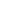### الصفحاتم1 الكهرباء مرحلة اولى

# اسئلة كهرباء/م1هندسة ميكانيك/لعام 2012

## نهائي دور اول 2012

University of Basrah - College of Engineering
Department of Mechanical Engineering3
Subject: Fundamental of Electrical Eng. Class: First Year Examiner: Sumer S.Hardan
Exam: Final 2012 Date: 11/06/2012 Time: 3 Hours
Attempt All Questions

Q1] (7 Marks)

A ring has a diameter of 21cm and a cross-sectional area of 10cm2. The ring is made up of semicircular sections of cast iron and cast steel, with each joint having a reluctance equal to an air-gap of 0.2mm. Find the ampere-turns required to produce a flux of 8x104 wb. The relative permeabilities of cast steel and cast iron are 800 and 166 respectively.

Q2] (7 Marks)

Two coils A and B are connected in series across a 240 V, 50 Hz supply. The resistance of A is 50 and inductance of B is 0.015H. If the input from the supply is 3kW and 2kVAR. Find the inductance of A and the resistance of B. Calculate the voltage across each coil.

Q3] (7 Marks)

Find the current in the 3 resistor by using Maxwell's currents.

Q4] (7 Marks)
An aluminium wire 7.5 m long is connected in parallel with a copper wire 6 m long. When a current of 5 A is passed through the combination, it is found that the current in aluminium wire is 3 A. The diameter of the aluminium wire is 1 mm. Determine the diameter of the copper wire. Resistivity of copper is 0.017u-m; that of the aluminium is
الوجه الاول(Q5] (7 Marks

Calculate the current passing through the 150 resistor by using Thevenin's theorem.

Q6) (5 Marks)

Calculate the equivalent resistance Rab at terminal a-b07] (5 Marks)

Calculate the RMS value of the voltage waveform that shown in figure below.

الوجه الثاني.
.
.

### الفصل الثاني 2012

.

.University of Basrah

Engineering College Mechanical Department
Second seasonal Examiner: Sumer S.Hardan
Subject: Fundamental of Electrical Eng Stage
: First Year
Time: 1.5 Hours
Date: 21/4/2012

Second seasonal Examination for

year 2011-2012

Q1) Explain the following in briefly

1. Phase Difference.

2. Form Factor for sinusoidal waveform =1.11.

3. Power Factor correction.

(20) Marks

Q2] Find the waveform of the voltage v(t) across the current source in the circuit shown below given that e(t)=2.828 sin(5000t) V and i(t)=1.414 cos(10000t)A

Q3] For the circuit shown below, calculate the maximum power that can be transferred to Z

Q4] For the circuit shown below, calculate the current flowing through the load connected across terminals A and B byth.ven w.
.
.
اسألكم الدعــــــــــــــــــــــــــــــــــــــــــــــاءمهندس ميكانيك
هدفي هو التطوير من ذاتي ثم الاستفادة ثم تطوير غيري